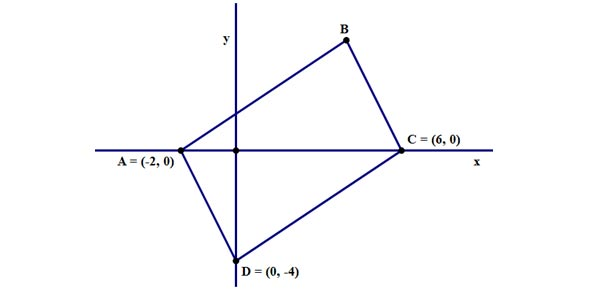# Coordinate Geometry-period 1

27 Questions | Total Attempts: 56SettingsThis is your chapter test on Coordinate Geometry.

Related Topics
• 1.
What is the point below?
• A.

(-2, 5)

• B.

(5, 2)

• C.

(5, -2)

• D.

(2, 5)

• 2.
What is the y-intercept of the graph below?
• A.

(0, 3)

• B.

(3, 0)

• C.

(1, 0)

• D.

(0, 1)

• 3.
What is the x-intercept of the graph below?
• A.

(0, 1)

• B.

(1, 0)

• C.

(0, -2)

• D.

(-2, 0)

• 4.
What is the midpoint of the two points: (-4, 3) and (2, 5)?
• A.

(-1, 4)

• B.

(-.5, 3.5)

• C.

(-3, -1)

• D.

(-2, 8)

• 5.
What is the midpoint between the two points below?
• A.

(2.5, -1)

• B.

(2, 1.5)

• C.

(0, 1.5)

• D.

(2.5, 1.5)

• 6.
What is the distance between the two points: (3, 5) and (-2, 4)?
• A.

5.10

• B.

6.32

• C.

1.41

• D.

5.61

• 7.
What is the distance between the two points below?
• A.

7.81

• B.

3.16

• C.

4.23

• D.

3.61

• 8.
What is the slope of the line with equation:  2y=6x+12
• 9.
What is the slope of a line with equation: y-3=2/3(x+5)
• 10.
What is the x-intercept of the line with equation: y+6=3(x+6)?
• A.

(-4, 0)

• B.

(6, 0)

• C.

(3, 0)

• D.

(-8, 0)

• 11.
What is the y-intercept of the line with equation: y-4=2(x-3)
• A.

(0, -3)

• B.

(0, -2)

• C.

(0, 2)

• D.

(-4, 0)

• 12.
What is the slope between the two points: (-7, 3) and (2, 9)?
• A.

6/ 5

• B.

2/3

• C.

-10/7

• D.

9/6

• 13.
What is the slope between the two points below?
• A.

1/2

• B.

-1/2

• C.

-2

• D.

1/7

• 14.
If a line has a slope of 3 and a y-intercept of (0, 4), what is the equation of the line?
• A.

Y=3x+4

• B.

Y=3(x-4)

• C.

Y=4x+3

• D.

Y-3=x-4

• 15.
What is the equation of a line with a slope of 1/4 and goes through the point (7, -3)?
• A.

Y-3=1/4(x-7)

• B.

Y=1/4x+3

• C.

Y+3=1/4(x-7)

• D.

Y=1/4(x-7)

• 16.
What is the slope of a line parallel to the line with equation: y=3/4x-8?
• A.

3/4

• B.

-4/3

• C.

8

• D.

-8

• 17.
What is the slope of a line perpendicular to the line with equation:  y-3=1/2(x+4)
• A.

-1/2

• B.

1/2

• C.

-2

• D.

2

• 18.
What is the equation of a line that is parallel to y=4x-2 and goes through the point (3, -7)?
• A.

Y=-1/4x-7

• B.

Y=4x-7

• C.

Y-7=4(x-3)

• D.

Y+7=4(x-3)

• 19.
What is the equation of a line that is perpendicular to the line with equation y-3=-4/5(x-2) and goes through the point (3, -5)?
• A.

Y=5/4x-5

• B.

Y+5=5/4(x-3)

• C.

Y=-4/5x-5

• D.

Y-3=5/4x+3

• 20.
What is the radius of a circle with equation: x^2 +y^2 =36
• 21.
What is the equation of the circle below?
• A.

(x-3)^2 + (y-3)^2 = 9

• B.

X^2+ y^2 = 3

• C.

X^2 + y^2 = 9

• D.

X^2 + (y-3)^2 = 9

• 22.
What is the center of the circle with equation: (x-2)^2 + (y+6)^2 = 49
• A.

(2, -6)

• B.

(0, 0)

• C.

(-2, 6)

• D.

(0, 7)

• 23.
What is the equation of the circle below?
• A.

X^2 + y^2 = 4

• B.

(x-3)^2 + (y+1)^2 = 4

• C.

(x+3)^2 + (y-1)^2 = 4

• D.

(x-2)^2 + (y-2)^2 = 4

• 24.
What is the equation of this line: 3y=x+9 in standard slope-intercept form?
• A.

Y=1/3x+3

• B.

Y=x+6

• C.

Y=x+3

• D.

Y=1/3x+9

• 25.
What is the equation of the line y-4=2(x-3) in standard slope-intercept form?
• A.

Y=2x

• B.

Y=2x-4

• C.

Y=2x-3

• D.

Y=2x-2